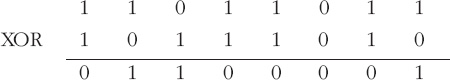Representing and manipulating data in computers

Start this free course now. Just create an account and sign in. Enrol and complete the course for a free statement of participation or digital badge if available.

Free course

# 8.5 The exclusive-OR operation

The exclusive-OR operation (usually abbreviated to XOR, pronounced ‘ex-or’) combines two binary words, bit by bit, according to the rules:

• 0 XOR 0 = 0

• 0 XOR 1 = 1

• 1 XOR 0 = 1

• 1 XOR 1 = 0

In other words, the result is 1 when either bit is 1 but not when both bits are 1 or both bits are 0, or the result is 1 when the two bits are different and 0 when they are the same.

## Example 12

Find the result of 1101 1011 XOR 1011 1010.

The bits in the two words are combined according to the above rules, working along the two words. For instance, the rightmost bit of the result is derived from 1 XOR 0=1. Doing this for all the bits gives:so the result is 0110 0001.